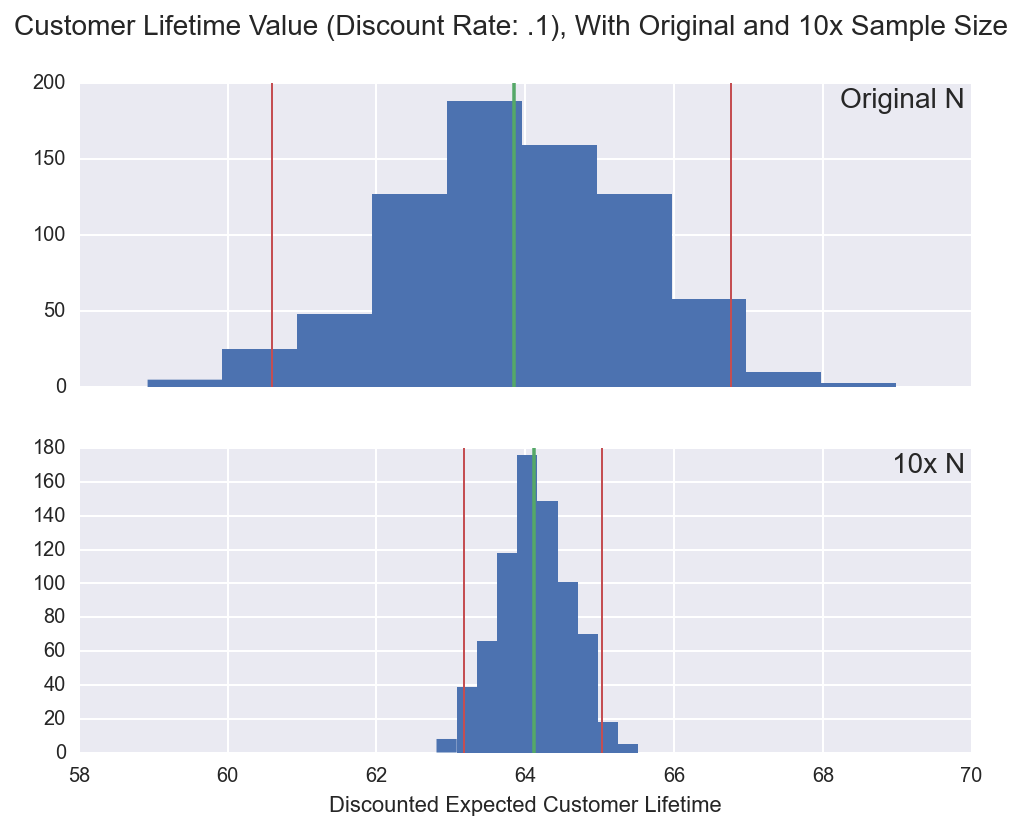# Using MCMC to Fit the Shifted-Beta-Geometric Customer Lifetime Value Model

Two professors of marketing, Peter Fader and Bruce Hardie, have developed probability models for estimating customer lifetime value (LTV). In their papers and example spreadsheets, they estimate the models using maximum-likelihood estimation (MLE).

In this post, I'm going to show how to use MCMC (via pymc) to estimate one of the models they've developed. Using MCMC makes it easy to quantify the uncertainty of the model parameters, and because LTV is a function of the model parameters, to pass that uncertainty through into the estimates of LTV itself.

This post is primarily about implementing the model, and I'm only going to touch briefly on the strengths of the Fader/Hardie model over simpler, back-of-the-envelope formulas you'll find if you google 'calculate customer lifetime value.' But in the interest of motivating the implementation, the model is worth understanding because:

1. by modeling the processes underlying aggregate metrics like 'churn rate' or 'repeated buying rate,' and
2. by allowing for heterogeneity in a customer base,

it provides more insight into customer behavior and in many cases, will provide less biased predictions about future behavior of customers.

# The Four LTV Scenarios¶

The academic literature on LTV breaks down the problem into four scenarios, depending on whether the customer-business relationship is contractual, and on whether customers have discrete opportunities for transactions. The following graphic, taken from the second paper listed below, provides examples for each of the four scenarios.

In :
import os
from IPython.display import Image, HTML
CHART_DIR = os.path.join(os.getcwd(), 'charts/')
Embed = Image(CHART_DIR + 'quadrant.png', retina=True)
Embed

Out: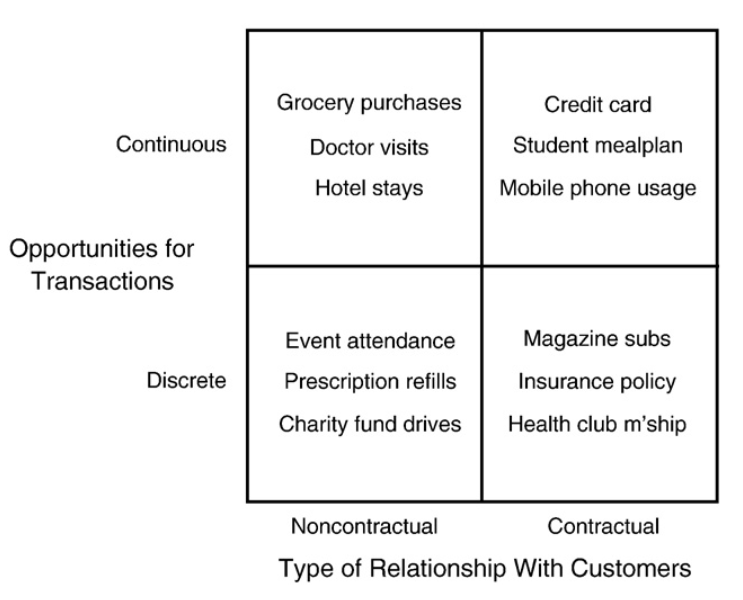The key distinction between contractual and noncontractual is that in contractual scenario, you observe when a customer leaves, while in a noncontractual scenario, customer departure is unobservable and therefore must be inferred. In this post, I'm going to implement a model from the contractual-discrete (lower-right) quadrant: the shifted-beta-geometric (sBG) model.

The paper introducing the sBG model is:

• Peter S. Fader & Bruce G.S. Hardie, "How to Project Customer Retention." Journal of Interactive Marketing, 21 (2007), 76–90. link

If you're interested in learning more about the larger world of probability models in LTV estimation, this paper provides a nice overview:

• Peter S. Fader & Bruce G.S. Hardie, "Probability Models for Customer-Base Analysis." Journal of Interactive Marketing, 23 (2009), 61–69. link

# Contractual-Discrete: The sBG Model¶

The shifted-beta-geometric (sBG) model makes two assumptions:

1. Each customer has a constant churn probability $\theta$. You can think of this as the customer flipping a weighted coin (with probability of tails = $\theta$) at the end of every subscription period, and they cancel their membership if the coin lands tails.
2. Customer churn probabilities are drawn from a beta distribution with parameters $\alpha$ and $\beta$.

The strength and flexibility of the model comes from the second assumption, which allows for heterogeneity in customer churn rates. In contrast, simple LTV formulas usually assume a constant aggregate churn rate. If you've worked with subscription data, this assumption probability matches your intuition: maybe you've got some lifers who seem like they'll renew until the end of time, and some short-lifers who try the product for a period or two and then cancel. While this heterogeneity can be approximated by the constant aggregate churn rate used in simpler LTV formulas, the aggregate churn rate often results in downwardly-biased estimates of LTV. (See the 'Perils of Ignoring Heterogeneity' paper linked to below for more on this bias.)

Estimating the sBG model amounts to finding $\alpha$ and $\beta$, effectively estimating what proportion of your customer base is lifers, what proportion short-lifers, and what proportion fall on the spectrum in between. Using Matplotlib, we can recreate figure 3 from the sBG paper, illustrating how the beta distribution permits a variety of churn-rate distribution shapes - and thus how the sBG model can fit a variety of customer bases.

In :
%matplotlib inline
%config InlineBackend.figure_format = 'retina'

In :
import numpy as np
import scipy
import pymc as pm
import seaborn as sns
import pandas as pd
import matplotlib.pyplot as plt

In :
sns.set(style="white")
current_palette = sns.color_palette()
fig, ax = plt.subplots(figsize=(8,6))
ax.set_ylim(0, 2)
ax.set_xlim(0, 2)
x = np.linspace(0, 1, 100)[1:99]
subplot_locations = [[.6, .61, .25, .25], [.6, .21, .25, .25], [.2, .61, .25, .25], [.2, .21, .25, .25]]
subplot_params = [(2, 7), (1.3, .7), (.7, 1.3), (.5, .5)]
for loc, params in zip(subplot_locations, subplot_params):
ax_i = plt.axes(loc)
ax_i.plot(x, scipy.stats.beta.pdf(x, params, params), '-')
plt.setp(ax_i.get_yticklabels(), visible=False)
ax_i.axes.spines['right'].set_color('none')
ax_i.axes.spines['top'].set_color('none')
ax_i.set_xlabel(r'$\theta$')
ax_i.set_xticks([0., .5, 1])

ax.set_title(r'General Shapes of the Beta Distribution as a Function of $\alpha$ and $\beta$')
ax.axes.spines['right'].set_color('none')
ax.axes.spines['top'].set_color('none')
ax.plot([1, 1], ax.get_ylim(), '--', c='black', lw=1)
ax.plot(ax.get_xlim(), [1, 1], '--', c='black', lw=1)
ax.set_xticks([0., 1., 2.])
ax.set_yticks([0., 1., 2.])
ax.set_xticklabels(['0', '1', r'$\alpha$'])
_ = ax.set_yticklabels(['0', '1', r'$\beta$'])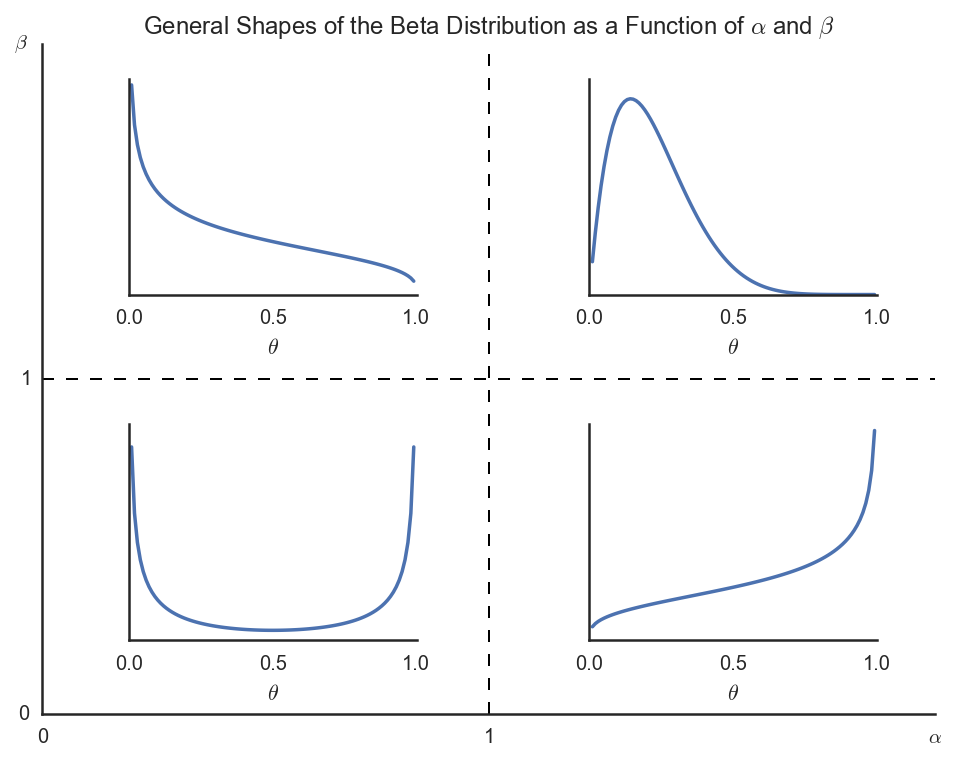(If anyone can reproduce this using plt.subplots() or plt.subplot2grid(), I'd love to know how.)

In their 2007 paper, Fader and Hardie demonstrate how to fit as sBG model to data using MLE in Excel. They begin by deriving the likelihood function, then demonstrate how to code it in Excel and how to use Excel's solver to maximize the likelihood function with respect to $\alpha$ and $\beta$.

To fit the model using MCMC and pymc, we'll take the likelihood function they derived, code it in Python, and then use MCMC to sample from the posterior distributions of $\alpha$ and $\beta$. This post is more about implementation than derivation, so I'll just explain the intuition of the likelihood function without going into the details of the derivation.

I'll use the example data they use in Appendix B. These are counts of active users in period 0, period 1, etc.

In :
example_data = [1000, 869, 743, 653, 593, 551, 517, 491]


To calculate the likelihood, we'll need the number of customers lost at each period.

In :
def n_lost(data):
lost = [None]
for i in range(1, len(data)):
lost.append(data[i - 1] - data[i])
return lost

example_data_n_lost = n_lost(example_data)

data = (example_data, example_data_n_lost)
data

Out:
([1000, 869, 743, 653, 593, 551, 517, 491],
[None, 131, 126, 90, 60, 42, 34, 26])

Note that I've smushed the two series (counts of active, and lost per period) into a single tuple. I've done this so I can pass the data to pymc as a single object.

I'll define uninformative priors on $\alpha$ and $\beta$.

In :
alpha = pm.Uniform('alpha', 0.00001, 1000, value=1)
beta = pm.Uniform('beta', 0.00001, 1000, value=1)


And now we start getting into the meat and potatoes of the likelihood function, which includes two components:

• the probability that $n_i$ customers churn in period $i$ (in this case, we observe $i$ up to 7)
• the probability that $n - \sum\limits_{t=1}^7n_t$ customers are still active at the end of the 7th period.

To help us with the first component, we need the probability that a randomly chosen individual will have a lifetime $T = t$ given $\alpha$ and $\beta$, equation (7) in the paper:

In :
num_periods = len(example_data)

@pm.deterministic
def P_T_is_t(alpha=alpha, beta=beta, num_periods=num_periods):
p = [None, alpha / (alpha + beta)]
for t in range(2, num_periods):
pt = (beta + t - 2) / (alpha + beta + t - 1) * p[t-1]
p.append(pt)
return p


"pm.deterministic" tells pymc that the output is completely (derministically) determined by the inputs, as is the case here.

For the second component of the likelihood function, we need the probability of a randomly chosen individual surviving to period $t$, given $\alpha$ and $\beta$:

In :
@pm.deterministic
def survival_function(P_T_is_t=P_T_is_t, num_periods=num_periods):
s = [None, 1 - P_T_is_t]
for t in range(2, num_periods):
s.append(s[t-1] - P_T_is_t[t])
return s


We're now ready to define the likelihood function itself. We can see the two components described above:

In :
@pm.observed
def retention_rates(P_T_is_t=P_T_is_t, survival_function=survival_function, value=data):
def logp(value, P_T_is_t, survival_function):

active, lost = value

# Those who've churned along the way...
died = np.log(P_T_is_t[1:]) * lost[1:]

# and those still active in last period
still_active = np.log(survival_function[-1]) * active[-1]
return sum(died) + still_active


"pm.observed" tells pymc that we've observed this variable's value, so it's fixed.

To make sure that we've coded everything correctly, we can confirm that our log likelihood matches that in the paper (when $\alpha = 1$ and $\beta = 1$):

In :
mcmc = pm.MCMC([alpha, beta, P_T_is_t, survival_function, retention_rates])
retention_rates.logp

Out:
-2115.545506645605

Yep, it matches (Figure B1 in the paper).

At this point, we can estimate the posterior distributions of $\alpha$ and $\beta$ using MCMC:

In :
mcmc.sample(20000, 5000, 20)

 [-----------------100%-----------------] 20000 of 20000 complete in 11.4 sec

...and plot the posterior distribution using pymc's built-in plot method:

In :
sns.set(style="darkgrid")
pm.Matplot.plot(alpha)

Plotting alpha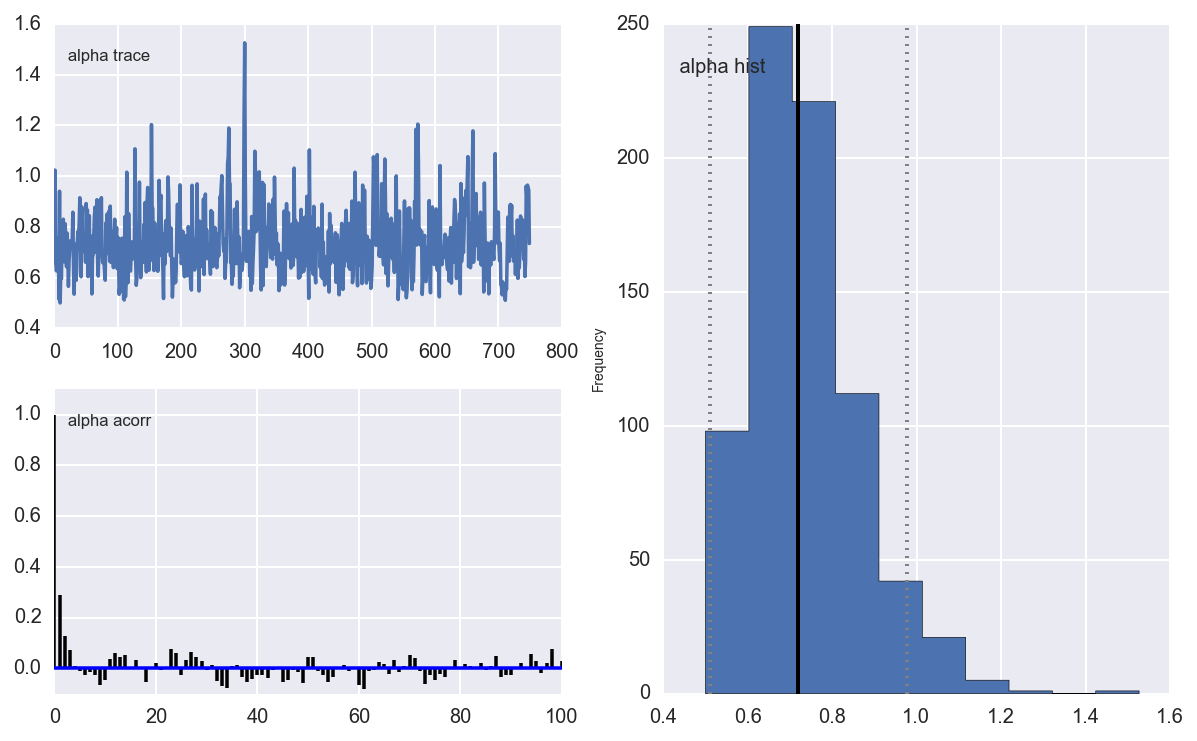In :
pm.Matplot.plot(beta)

Plotting beta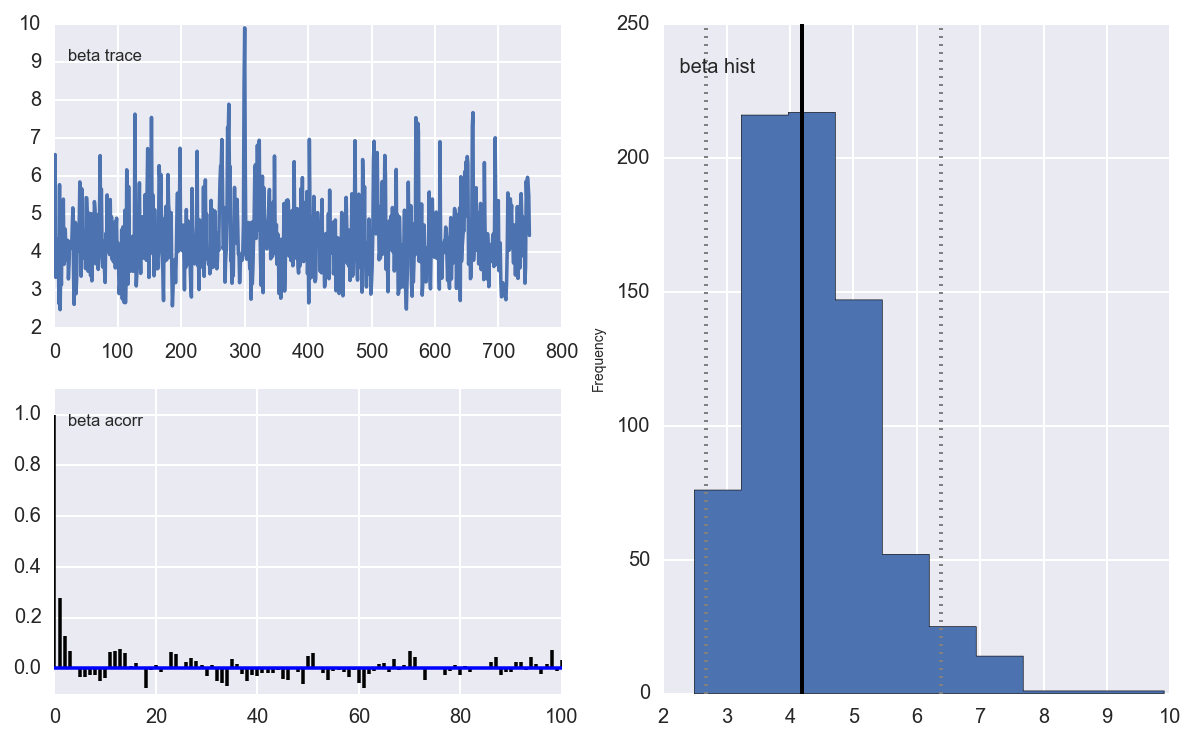It looks like the medians of the posterior distributions are close to the MLE estimates Fader and Hardie found (.668 for $\alpha$ and 3.806 for $\beta$). Let's confirm:

In :
df_trace = pd.DataFrame({'alpha': alpha.trace(), 'beta': beta.trace()})
df_trace.median()

Out:
alpha    0.717973
beta     4.188330
dtype: float64

So why go through the trouble of using MCMC over MLE? Because now we have an easy way to understand the uncertainty of our estimates, without having to go through extra step of generating sampling distributions computing p values.

To demonstrate, I'm going to introduce a concept from another Fader/Hardie paper, the Discounted Expected Residual Lifetime (DERL).

The motivation for the DERL is that once you've fit an sBG model to a customer base, an obvious follow up question is, "how much money can I expect to take in from this customer base in the future?" The DERL for a customer who pays \x$per period and who is at the end of their$n$period is the number such that$DERL * x$is the expected present value of future payments from that customer. The DERL is a function of$\alpha$,$\beta$, a discount rate$d$, and the current period$n$of the subscriber. The DERL can also give us the expected discounted CLV of a new customer, if we set$n = 1$and add an undiscounted initial payment. And because we have posterior distributions for$\alpha$and$\beta$, we can easily leverage our uncertainty in$\alpha$and$\beta$to understand our uncertainty in the statistic we really care about, the CLV. The paper deriving the DERL is: • Peter S. Fader & Bruce G.S. Hardie, "Customer-Base Valuation in a Contractual Setting: The Perils of Ignoring Heterogeneity" Marketing Science, 29-1 (Jan-Feb 2010), 85-93. link Let's start by defining function to compute the DERL: In : from scipy.special import hyp2f1 def derl(alpha, beta, d, n): """ Discounted Expected Residual Lifetime, as derived in Fader and Hardie (2010). See equation (6). :param alpha: sBG alpha param :param beta: sBG beta param :param d: discount rate :param n: customer's contract period (customer has made n-1 renewals) :return: float """ return (beta + n - 1) / (alpha + beta + n - 1) * hyp2f1(1, beta + n, alpha + beta + n, 1 / (1 + d))  For each draw of the posterior distribution, we'll calculate the DERL for a customer just before their first renewal decision. For the sake of example, imagine we get$10 per period from each customer, and I'll use a discount rate of 10%.

In :
df_trace['derl'] = df_trace.apply(lambda x: 1 + derl(x['alpha'], x['beta'], .1, 1), axis=1)


Now we can provide not only an estimate of discounted customer lifetime value, but also a credible interval:

In :
median_clv = df_trace.lifetime_value.median()
ax.set_title('Customer Lifetime Value (Discount Rate: .1)')
ax.plot([median_clv, median_clv], ax.get_ylim())
plt.annotate('Median: %.1f' % median_clv, xy=(median_clv + .02, ax.get_ylim()-10))
ax.plot([cred_interval, cred_interval], ax.get_ylim(), c=sns.color_palette(), lw=1)
_ = ax.plot([cred_interval, cred_interval], ax.get_ylim(), c=sns.color_palette(), lw=1)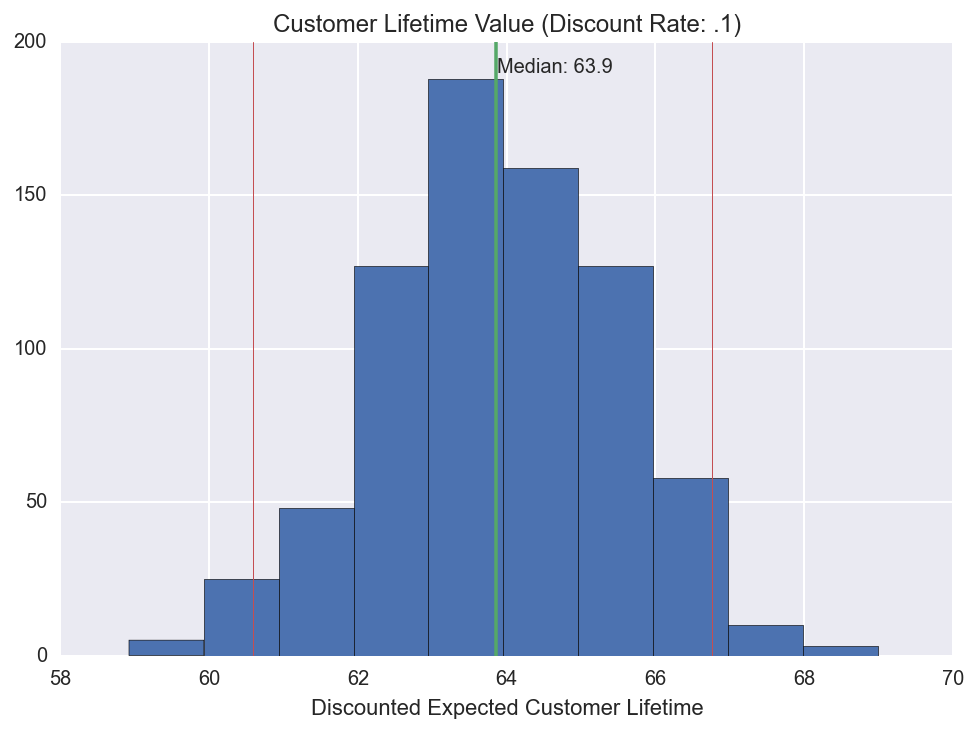As we get more data, our credible interval should shrink. Let's confirm by imagining we had 10x the data:

In :
example_data = [10 * x for x in example_data]
example_data_n_lost = n_lost(example_data)

data = (example_data, example_data_n_lost)
data

Out:
([10000, 8690, 7430, 6530, 5930, 5510, 5170, 4910],
[None, 1310, 1260, 900, 600, 420, 340, 260])

We have to redefine retention_rates so it knows about the new data:

In :
@pm.observed
def retention_rates(P_T_is_t=P_T_is_t, survival_function=survival_function, value=data):
def logp(value, P_T_is_t, survival_function):

active, lost = value

# Those who've churned along the way...
died = np.log(P_T_is_t[1:]) * lost[1:]

# and those still active in last period
still_active = np.log(survival_function[-1]) * active[-1]
return sum(died) + still_active


Re-run MCMC....

In :
mcmc = pm.MCMC([alpha, beta, P_T_is_t, survival_function, retention_rates])
mcmc.sample(20000, 5000, 20)
df_trace_10x = pd.DataFrame({'alpha': alpha.trace(), 'beta': beta.trace()})
df_trace_10x['derl'] = df_trace_10x.apply(lambda x: 1 + derl(x['alpha'], x['beta'], .1, 1), axis=1)

 [-----------------100%-----------------] 20000 of 20000 complete in 15.4 sec

... and plot our two posterior distributions on the same X axis:

In :
f, (ax1, ax2) = plt.subplots(2, 1, sharex=True, figsize=(8, 6))

plots = [(ax1, df_trace, 'Original N'), (ax2, df_trace_10x, '10x N')]
for ax, df, title in plots: